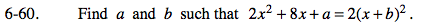### Home > PC > Chapter 6 > Lesson 6.1.4 > Problem6-60

6-60.Expand the right side of the equation.

Subtract 2x2 from both sides of the equation.

One solution would be if 8x equaled 4bx and
a equaled 2b2.

2x2 + 8x + a = 2x2 + 4bx + 2b2

8x + a = 4bx + 2b2

b = 2 and a = 8